# Fraction Exponent Calculator

Created by Hanna Pamuła, PhD candidate
Reviewed by Bogna Szyk and Jack Bowater
Last updated: Jun 05, 2020

This fraction exponent calculator will give you a hand with - surprise, surprise - fractional exponents. Do you struggle with the concept of fractional exponents? Are negative and fractional exponents are a closed book to you? Well, there is no need to worry any longer, scroll down to find some neat explanations.

## Fractional exponents with the numerator equal to 1

The fractional exponents are a way of expressing powers as well as roots in one notation.

What does it mean, exactly? Let's have a look at a few simple examples first, where our numerator is equal to 1:

• 64 (1/2) = √64
• 27 (1/3) = ³√27

From the equations above we can deduce that:

• An exponent of 1/2 is a square root
• An exponent of 1/3 is a cube root
• An exponent of 1/4 is a fourth root
• ...
• An exponent of 1/k is a k-th root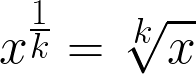But why is it so? We'll try to prove it:
1. Let's use the law of exponents which says that we can add the exponents when multiplying two powers that have the same base:

xa+b = xa * xb

so, for example, if n = 2

x² = x¹⁺¹ = x¹ * x¹ = x * x

Try this with any number you like, it's always true!

2. Then, let's look at the fractional exponents of x:

x = x¹ = x (1/2 + 1/2) = x (1/2) * x (1/2)

How do you call a number which - when multiplied by itself - gives another number? It's a square root, of course! So we found out that:

x (1/2) = √x

3. If you like, you can analogically check other roots, e.g. the cube root:

x = x (1/3 + 1/3 + 1/3) = x (1/3) * x (1/3) * x (1/3) = ³√x * ³√x * ³√x

so

x (1/3) = ³√x

Now we know that x to the power of one third is equal to the cube root of x.

## Fractional exponents with a numerator different from 1 (any fraction)

So what happens if our numerator is not equal to 1 (n≠1)?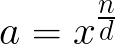All you need to do is to raise that number to that power n and take the d-th root. The order doesn't matter, as a fraction n/d may be split into two parts:

• whole number (n)
• a fraction part (1/d)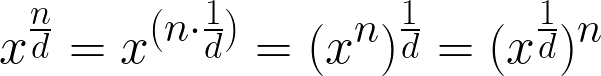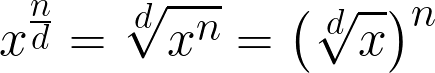Let's have a look at the example where the fractional exponent = 3/2 and x = 16:

• 16 3/2 = 16 (3 * 1/2) = (163)1/2 = √(16³) = √4096 = 64

Or, alternatively, we can write that

• 16 3/2 = 16 (1/2) * 3 = (161/2)3 = (√16)³ = 4³ = 64

And the result is the same, indeed. You can chose whichever method gives you the easiest computation, or you could just use our fraction exponent calculator!

## Negative and fractional exponents

Positive exponents tell us how many times we use a number in the multiplication: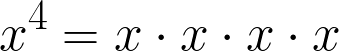But what happens if our exponent is a negative number, can you guess? Yes, it tells you how many times you need to divide by that number: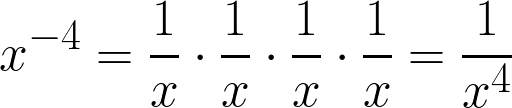Also, you can simply calculate the positive exponent (like x4) and then take the reciprocal of that value (1/x4 in our case). Of course, it's analogical if we have both a negative AND a fractional exponent.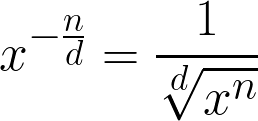## Fraction exponent calculator - how to use

We believe that the tool is so intuitive and straightforward, that no further explanation is needed, but just for the record we'll quickly explain how to calculate the fractional exponents:

1. Enter the base value. Let's type 7 for example.
2. Type the numerator and denominator of the fraction. If you want to use this calculator as a simple exponent tool - with an integer as the exponent, instead of a fraction - type 1 as the denominator. Assume our fraction is equal to -2/5. Enter -2 in the numerator and 5 in the denominator box (signs the other way round work as well).
3. Enjoy the result displayed by our fractional exponent calculator! It's 0.4592 for our exemplary problem.

Do we need to mention that our tool is flexible? You don't need to go from the top to the bottom of the calculator - calculate any unknown you want! Type any three values, and the fourth one will appear in no time.

Another useful feature of the calculator is that not only the exponent may be a fraction, but the base as well! For example, if you want to calculate (1/16) 1/2, just type 1/16 into base box. Awesome!

Hanna Pamuła, PhD candidateBase (x)
Numerator (n)
Denominator (d)
Fractional exponent (a)
People also viewed…

### Cosine

This cosine calculator helps to find out the cosine value for a specific angle in degrees or radians.

### Grams to cups

The grams to cups calculator converts between cups and grams. You can choose between 20 different popular kitchen ingredients or directly type in the product density.

### Manhattan distance

The Manhattan distance calculator is a simple calculator that determines the Manhattan distance (also known as the taxicab or city block distance) between two points in up to four-dimensional Cartesian space.

### Podcasts

Do you feel like you could be doing something more productive or educational while on a bus? Or while cleaning the house? Well, why don't you dive into the rich world of podcasts! With this podcast calculator, we'll work out just how many great interviews or fascinating stories you can go through by reclaiming your 'dead time'!